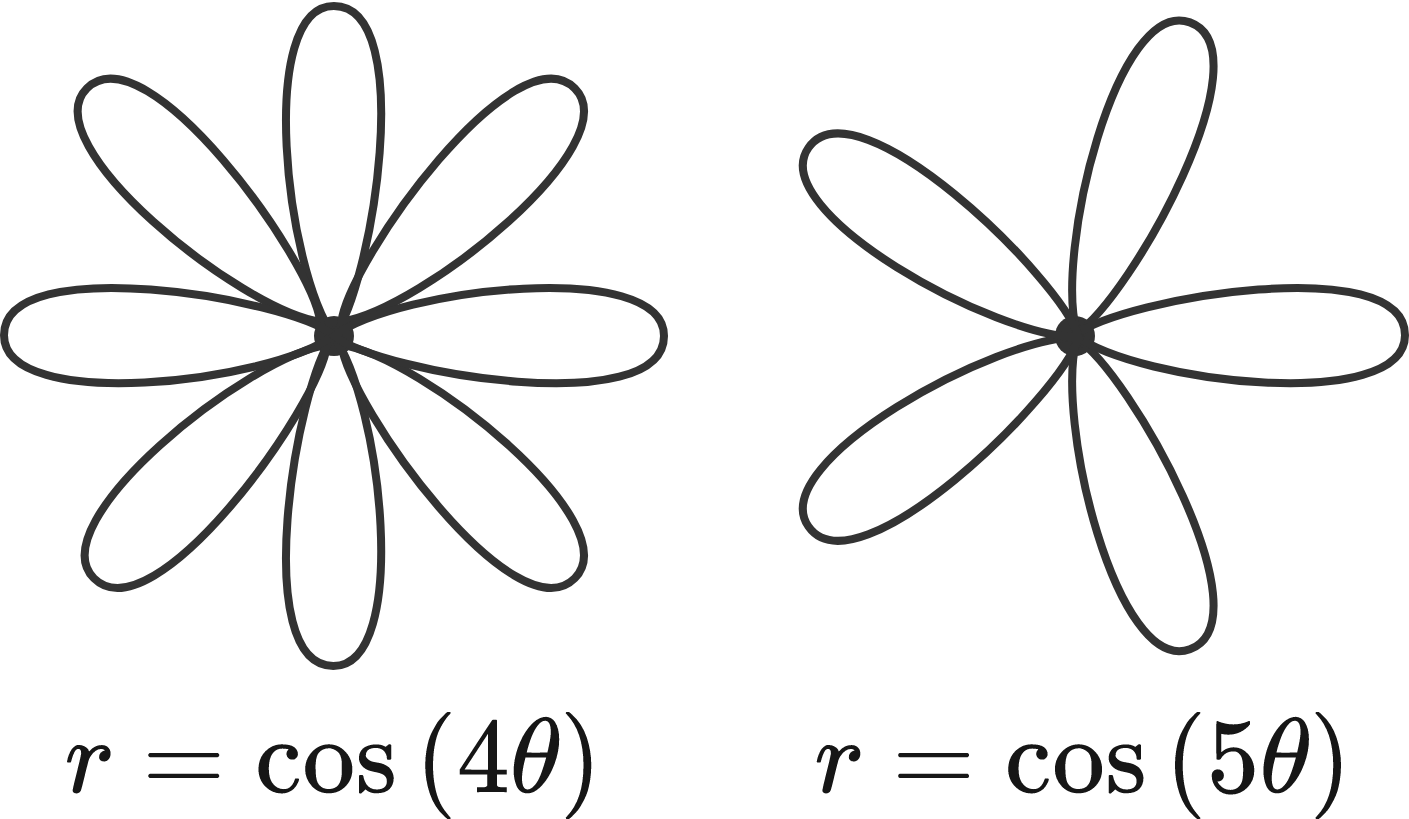# Rose Petals$P(k)$ is the number of petals in a rose formed by the polar equation $r = \cos(k\theta).$

For example, $P(4) = 8$ because $r = \cos(4\theta)$ has $8$ petals, and $P(5) = 5$ because $r = \cos(5\theta)$ has $5$ petals.

Is there a positive integer $k$ such that $P(k) = 2018?$

×